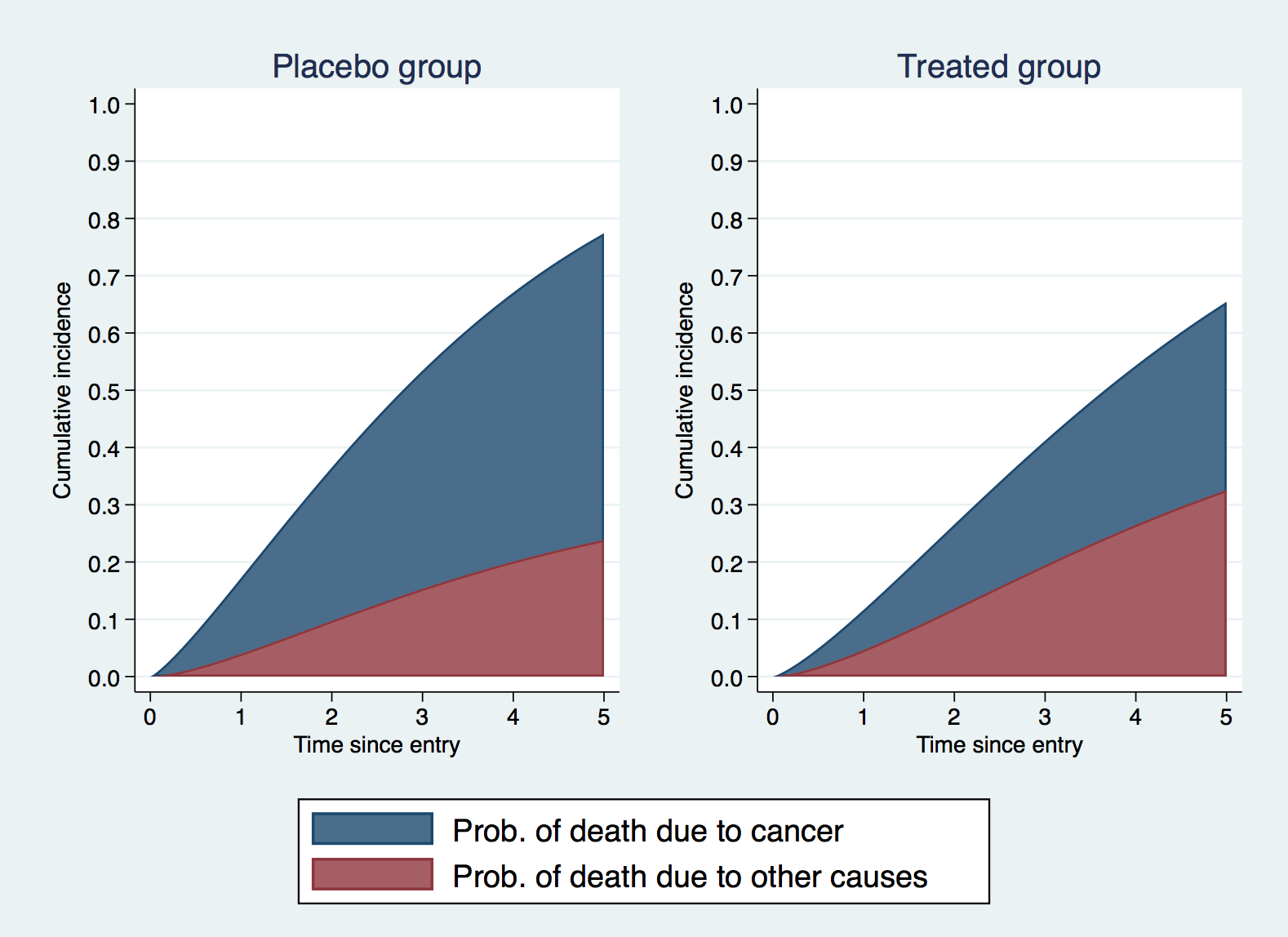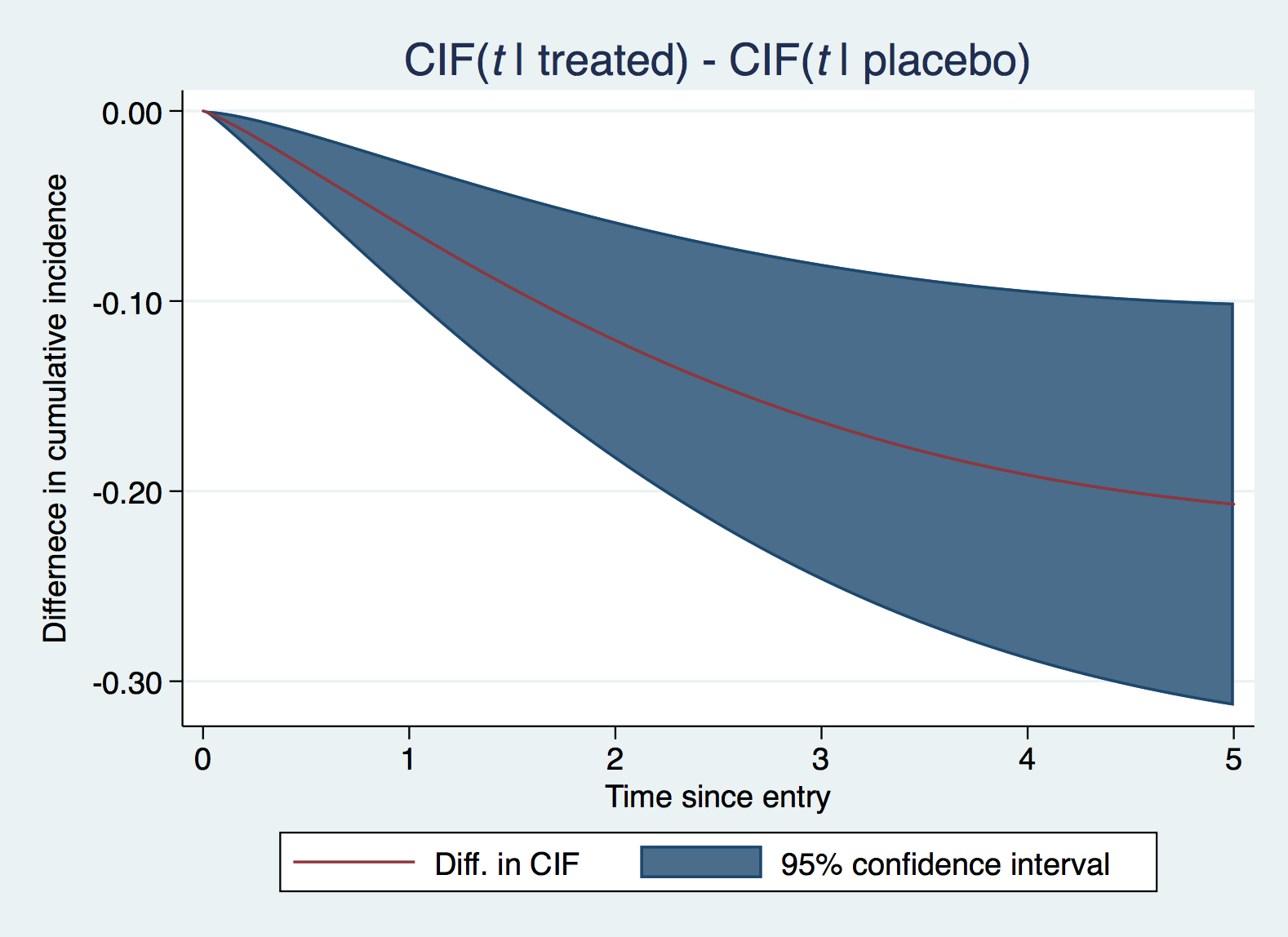Joint longitudinal and competing risks model

This one extends the standard joint longitudinal-survival model to account for competing risks, allowing the longitudinal outcome to be associated with each competing event. I’ll stick with a continuous longitudinal outcome,

$$y_{}(t) = m_{i}(t) + \epsilon_{ij}$$

where

$$m_{i}(t) = X_{1ij}(t)\mathbf{\beta}_{1} + Z_{ij}(t)b_{i}$$

and

$$b_{i} \sim N(0,\Sigma)$$

We now have $K$ competing events, each of which can be dependent on some (or many) aspects of the biomarker trajectory function, $m_{i}(t)$. To keep things simple, I’ll stick with the current value association structure, so we have for the $k$th competing risk,

$$h_{k}(t) = h_{0k}(t) \exp(X_{2ijk}\mathbf{\beta}_{2k} + \alpha_{k} m_{ik}(t))$$

where $\alpha_k$ is the log hazard ratio for the $k$th cause for a one-unit increase in the biomarker, at time $t$. Each competing event can be modelled however we like, with different baseline hazard functions, and with different covariate effects. Of course, we can also have different or additional association structures for each event.

To illustrate, I’m going to show you how to simulate data from a joint longitudinal and competing risks model, with two competing risks, representing death from cancer and death from other causes, and then show how to fit the true model using merlin.

Let’s assume a simple random intercept and random linear trend for the biomarker trajectory, i.e.

$$m_{i}(t) = (\beta_{10} + b_{0i}) + (\beta_{11} + b_{1i})t$$

We start by setting a seed, as always, and generate a sample of 300 patients and create an id variable.

. clear

. set seed 7254

. set obs 300
number of observations (_N) was 0, now 300

. gen id = _n



Next we generate our subject-specific random effects, for the intercept and slope. We need a single draw from each distribution, per patient. I’m assuming the random effects are independent, but that’s easily adapted using drawnorm. I also generate a treatment group indicator, assigning to each arm with 50% probability.

. gen b0 = rnormal(0,1)

. gen b1 = rnormal(0,0.5)

. gen trt = runiform()>0.5



Now we simulate cause-specific event times, using survsim. Simulating joint longitudinal-survival data is not the easiest of tasks mathematically, but thanks to survsim it’s pretty simple. We simply specify our cause-specific hazard function, and survsim does the hard work (numerical integration nested within root-finding), details in Crowther and Lambert (2013).

I specify my baseline distribution parameters, the scale and shape, for a Weibull baseline hazard for cause one, and an administrative censoring time of 5 years. I also specify a treatment effect with a log hazard ratio of -0.5, Now we call survsim, simulating under the current value association structure, defining $\alpha_{1}=0.2$:

. //cause one
. local maxt 5

. local l1 0.1

. local g1 1.2

. survsim t1 d1, hazard(l1':*g1':*#t:^(g1'-1) :* exp( 0.2 :* (b0 :+ (0.1:+b1):*#t))) ///
>                covariates(trt -0.5) maxtime(maxt')
Warning: 147 survival times were above the upper limit of 5
They have been set to 5 and can be considered censored
You can identify them by _survsim_rc = 3



which simulates our event times and stores them in t1, and censoring indicator in d1 given administrative censoring at 5 years.

Next we do the same, but for cause 2, again assuming a Weibull baseline hazard, but with $\alpha_{2}=-0.2$, and no treatment effect this time,

. //cause two
. local maxt 5

. local l2 0.05

. local g2 1.5

. survsim t2 d2, hazard(l2':*g2':*#t:^(g2'-1) :* exp(-0.2 :* (b0 :+ (0.1:+b1):*#t))) ///
>                maxtime(maxt')
Warning: 181 survival times were above the upper limit of 5
They have been set to 5 and can be considered censored
You can identify them by _survsim_rc = 3



Now we simply have to find the minimum between our simulated event times, and the censoring time of 5 years, as follows,

. egen double stime = rowmin(t1 t2)



and create our cause-specific event indicators,

. local maxt = 5

. replace d1 = 0

. replace d1 = 1 if t1==stime & stime!=maxt'

. replace d2 = 0

. replace d2 = 1 if t2==stime & stime!=maxt'



and we’re all done with the competing risk outcomes. Now onto our biomarker.

Let’s assume a setting where our biomarker is recorded at baseline, and then annually, so each patient can have up to five measurements. The easiest thing to do is to expand our dataset, by creating replicants of each row, and then drop any observations which occur after each patient’s event/censoring time.

. expand 5
(1,200 observations created)

. bys id : gen time = _n-1

. drop if time>stime
(460 observations deleted)



We generate our oberserved longitudinal biomarker measurements from the true model above,

. gen xb = b0 + 0.1 * time + b1 * time

. gen y = rnormal(xb,0.5)



It’s easiest to work in wide format for merlin, so for the competing risks outcomes we need to have only a single
row of data per patient, so we replace the repeated event times and event indicators with missings.

. bys id (time) : replace stime = . if _n>1
(740 real changes made, 740 to missing)

. bys id (time) : replace d1 = . if _n>1
(740 real changes made, 740 to missing)

. bys id (time) : replace d2 = . if _n>1
(740 real changes made, 740 to missing)



We’re now all set up to fit our data-generating, true model, with,

merlin (y fp(time, pow(1)) fp(time, pow(1))#M2[id]@1 M1[id]@1, family(gaussian) timevar(time)) ///
(stime trt EV[y], family(weibull, failure(d1)) timevar(stime))                          ///
(stime trt EV[y], family(weibull, failure(d2)) timevar(stime))


which I would say is rather elegantly simple to specify for such a complex model…you may disagree. I’m using the EV[y] element type to link the current value of the biomarker to each cause-specific hazard model, making sure to specify the timevar() options, so time is correctly handled. This is also why we must use the fp() element type to specify functions of time (or rcs() if you prefer splines). We can use stime as our outcome in both survival models but simply use the cause-specific event indicators so we get the correct contributions to the likelihood.

Let’s fit the model:

. merlin (y fp(time, pow(1)) fp(time, pow(1))#M2[id]@1 M1[id]@1, family(gaussian) timevar(time)) ///
>        (stime trt EV[y], family(weibull, failure(d1)) timevar(stime))                          ///
>        (stime trt EV[y], family(weibull, failure(d2)) timevar(stime))
variables created for model 1, component 1: _cmp_1_1_1 to _cmp_1_1_1
variables created for model 1, component 2: _cmp_1_2_1 to _cmp_1_2_1

Fitting fixed effects model:

Fitting full model:

Iteration 0:   log likelihood = -2524.0236
Iteration 1:   log likelihood = -2089.4886
Iteration 2:   log likelihood =  -2074.904
Iteration 3:   log likelihood = -2074.5405
Iteration 4:   log likelihood = -2074.5404

Mixed effects regression model                  Number of obs     =      1,040
Log likelihood = -2074.5404
------------------------------------------------------------------------------
|      Coef.   Std. Err.      z    P>|z|     [95% Conf. Interval]
-------------+----------------------------------------------------------------
y:           |
fp() |   .1730224   .0372887     4.64   0.000     .0999379    .2461068
fp()#M2[id] |          1          .        .       .            .           .
M1[id] |          1          .        .       .            .           .
_cons |   .0632891   .0683684     0.93   0.355    -.0707105    .1972886
sd(resid.) |   .5256804   .0164346                      .4944362    .5588988
-------------+----------------------------------------------------------------
stime:       |
trt |    -.67082   .1824517    -3.68   0.000    -1.028419   -.3132212
EV[] |   .1286913   .0554915     2.32   0.020     .0199299    .2374526
_cons |  -1.960073   .1634629   -11.99   0.000    -2.280454   -1.639692
log(gamma) |   .1569507    .079653     1.97   0.049     .0008337    .3130677
-------------+----------------------------------------------------------------
stime:       |
trt |   .1221402    .219983     0.56   0.579    -.3090185    .5532989
EV[] |  -.0799977   .0639881    -1.25   0.211     -.205412    .0454166
_cons |   -3.11913    .253006   -12.33   0.000    -3.615013   -2.623247
log(gamma) |   .4064408   .0950544     4.28   0.000     .2201376     .592744
-------------+----------------------------------------------------------------
id:          |
sd(M1) |   1.094679    .051729                      .9978456     1.20091
sd(M2) |   .5060782   .0297633                      .4509797    .5679083
------------------------------------------------------------------------------



The model fits beautifully…unsurprisingly.

Now we have our fitted model, generally of most interest within a competing risks analysis is both the cause-specific hazard ratios, which we get from our results table, and estimates of the cause-specific cumulative incidence functions.

We use merlin’s predict engine to help us with such things. When predicting something which depends on time, it’s often much easier to specify a timevar(), which contains timepoints at which to predict at, which subsequently makes plotting much easier.

. range tvar 0 5 500
(540 missing values generated)



Let’s now predict each cause-specific cumulative incidence function, in particular, I’ll predict marginal CIFs, so we can interpret them as population-average predictions. I’ll also use the at() option so we can investigate the effect of treatment.

. predict cif1, cif marginal outcome(2) at(trt 0) timevar(tvar)
(540 missing values generated)

. predict cif2, cif marginal outcome(2) at(trt 1) timevar(tvar)
(540 missing values generated)

. predict cif3, cif marginal outcome(3) at(trt 0) timevar(tvar)
(540 missing values generated)

. predict cif4, cif marginal outcome(3) at(trt 1) timevar(tvar)
(540 missing values generated)



For plotting purposes, we often create stacked plots of CIFs, and one way to do this is to add them appropriately together to draw the area under the curve, and then overlay one of the CIFs..

. gen totalcif1 = cif1 + cif3
(540 missing values generated)

. gen totalcif2 = cif2 + cif4
(540 missing values generated)



We now plot the stacked, marginal CIFs for those in the placebo group and those in the treated group, and combine (using grc1leg from SSC):

. twoway (area totalcif1 tvar)(area cif3 tvar), name(g1,replace)                          ///
>         xtitle("Time since entry") ytitle("Cumulative incidence")                       ///
>         title("Placebo group") legend(cols(1)                                           ///
>                 order(1 "Prob. of death due to cancer" 2 "Prob. of death due to other causes")) ///
>         ylabel(,angle(h) format(%2.1f)) ylabel(0(0.1)1) nodraw


. twoway (area totalcif2 tvar)(area cif4 tvar), name(g2,replace)      ///
>         xtitle("Time since entry") ytitle("Cumulative incidence")   ///
>         title("Treated group")                                      ///
>         legend(cols(1) order(1 "Prob. of death due to cancer"       ///
>         2 "Prob. of death due to other causes"))                    ///
>         ylabel(,angle(h) format(%2.1f)) ylabel(0(0.1)1) nodraw


. grc1leg g1 g2We could directly quantify the impact of treatment on each CIF by predicting the difference. To get confidence intervals, we use Stata’s powerful predictnl command, which takes a bit of time, but gives us something really useful.

. predictnl double difcif = predict(cif marginal outcome(2) at(trt 1) timevar(tvar)) ///
>                         - predict(cif marginal outcome(2) at(trt 0) timevar(tvar)) ///
>                         , ci(difcif_lci difcif_uci)
(540 missing values generated)
note: confidence intervals calculated using Z critical values


. twoway (rarea difcif_lci difcif_uci tvar)(line difcif tvar), name(g3,replace)      ///
>         xtitle("Time since entry") ytitle("Differnece in cumulative incidence")    ///
>         title("CIF({it:t} | treated) - CIF({it:t} | placebo)")                     ///
>         ylabel(,angle(h) format(%3.2f))                                            ///
>         legend(order(2 "Diff. in CIF" 1 "95% confidence interval"))So the joint model I’ve talked about is an introductory example in the competing risks setting. Given merlin’s capabilities, it’s rather simple to extend to using other associations structures, as such the rate of change (dEV[y]) or the integral (iEV[y]) of the trajectory function, and to use different distributions for each cause-specific hazard model…the list of extensions goes on.